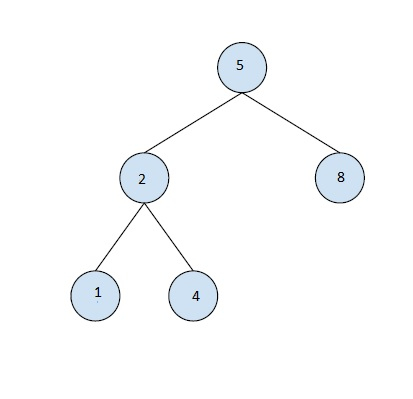# Find the largest BST subtree in a given Binary Tree - Set 1 in C++

In this problem, we are given a binary tree BT. Our task is to find the largest BST subtree in a given Binary Tree.

Binary Tree is a special data structure used for data storage purposes. A binary tree has a special condition that each node can have a maximum of two children.

Binary Search Tree (BST)is a tree in which all the nodes follow the below-mentioned properties −

• The value of the key of the left sub-tree is less than the value of its parent (root) node's key.

• The value of the key of the right subtree is greater than or equal to the value of its parent (root) node's key.

Let's take an example to understand the problem,

Input :Output : 3

Explanation

Full binary tree is a BST.

## Solution Approach

A simple solution to the problem is to perform in-order traversal of the tree. And for each node of the tree, check if its subtrees are BST or not. At last return the size of the largest subtree which is a BST.

## Example

Program to illustrate the working of our solution

#include<bits/stdc++.h>
using namespace std;
class node{
public:
int data;
node* left;
node* right;
node(int data){
this->data = data;
this->left = NULL;
this->right = NULL;
}
};
int findTreeSize(node* node) {
if (node == NULL)
return 0;
else
return(findTreeSize(node->left) + findTreeSize(node->right) + 1);
}
int isBSTree(struct node* node) {
if (node == NULL)
return 1;
if (node->left != NULL && node->left->data > node->data)
return 0;
if (node->right != NULL && node->right->data < node->data)
return 0;
if (!isBSTree(node->left) || !isBSTree(node->right))
return 0;
return 1;
}
int findlargestBSTSize(struct node *root) {
if (isBSTree(root)){
return findTreeSize(root);
}
else
return max(findlargestBSTSize(root->left), findlargestBSTSize(root->right));
}
int main() {
node *root = new node(5);
root->left = new node(2);
root->right = new node(8);
root->left->left = new node(1);
root->left->right = new node(4);
cout<<"The size of the largest possible BST is "<<findlargestBSTSize(root);
return 0;
}

## Output

The size of the largest possible BST is 5

Another Approach

Another solution to the problem is by traversing the tree from bottom and checking if it is BST using its child nodes. For this the node, we will keep track for

If it is a BST or not.

• The value of maximum element, in case of left subTree.

• Minimum element, in case of right subTree. These values need to be compared with the current node for the check of BST.

Also, the size of the largest BST will be updated by checking with the current BST size.

## Example

#include<bits/stdc++.h>
using namespace std;
class node{
public:
int data;
node* left;
node* right;
node(int data){
this->data = data;
this->left = NULL;
this->right = NULL;
}
};
int findlargestBSTSizeRec(node* node, int *minValRsubTree, int *maxValLsubTree, int *maxBSTSize, bool *isBSTree) {
if (node == NULL){
*isBSTree = true;
return 0;
}
int min = INT_MAX;
bool left_flag = false;
bool right_flag = false;
int leftSubtreeSize,rightSubTreeSize;
*maxValLsubTree = INT_MIN;
leftSubtreeSize = findlargestBSTSizeRec(node->left, minValRsubTree, maxValLsubTree, maxBSTSize, isBSTree);
if (*isBSTree == true && node->data > *maxValLsubTree)
left_flag = true;
min = *minValRsubTree;
*minValRsubTree = INT_MAX;
rightSubTreeSize = findlargestBSTSizeRec(node->right, minValRsubTree, maxValLsubTree, maxBSTSize, isBSTree);
if (*isBSTree == true && node->data < *minValRsubTree)
right_flag = true;
if (min < *minValRsubTree)
*minValRsubTree = min;
if (node->data < *minValRsubTree)
*minValRsubTree = node->data;
if (node->data > *maxValLsubTree)
*maxValLsubTree = node->data;
if(left_flag && right_flag){
if (leftSubtreeSize + rightSubTreeSize + 1 > *maxBSTSize)
*maxBSTSize = (leftSubtreeSize + rightSubTreeSize + 1);
return (leftSubtreeSize + rightSubTreeSize + 1);
}
else{
*isBSTree = false;
return 0;
}
}
int findlargestBSTSize(node* node){
int min = INT_MAX;
int max = INT_MIN;
int largestBSTSize = 0;
bool isBST = false;
findlargestBSTSizeRec(node, &min, &max, &largestBSTSize, &isBST);
return largestBSTSize;
}
int main(){
node *root = new node(5);
root->left = new node(2);
root->right = new node(8);
root->left->left = new node(1);
root->left->right = new node(4);
cout<<"The Size of the largest BST is "<<findlargestBSTSize(root);
return 0;
}

## Output

The Size of the largest BST is 5